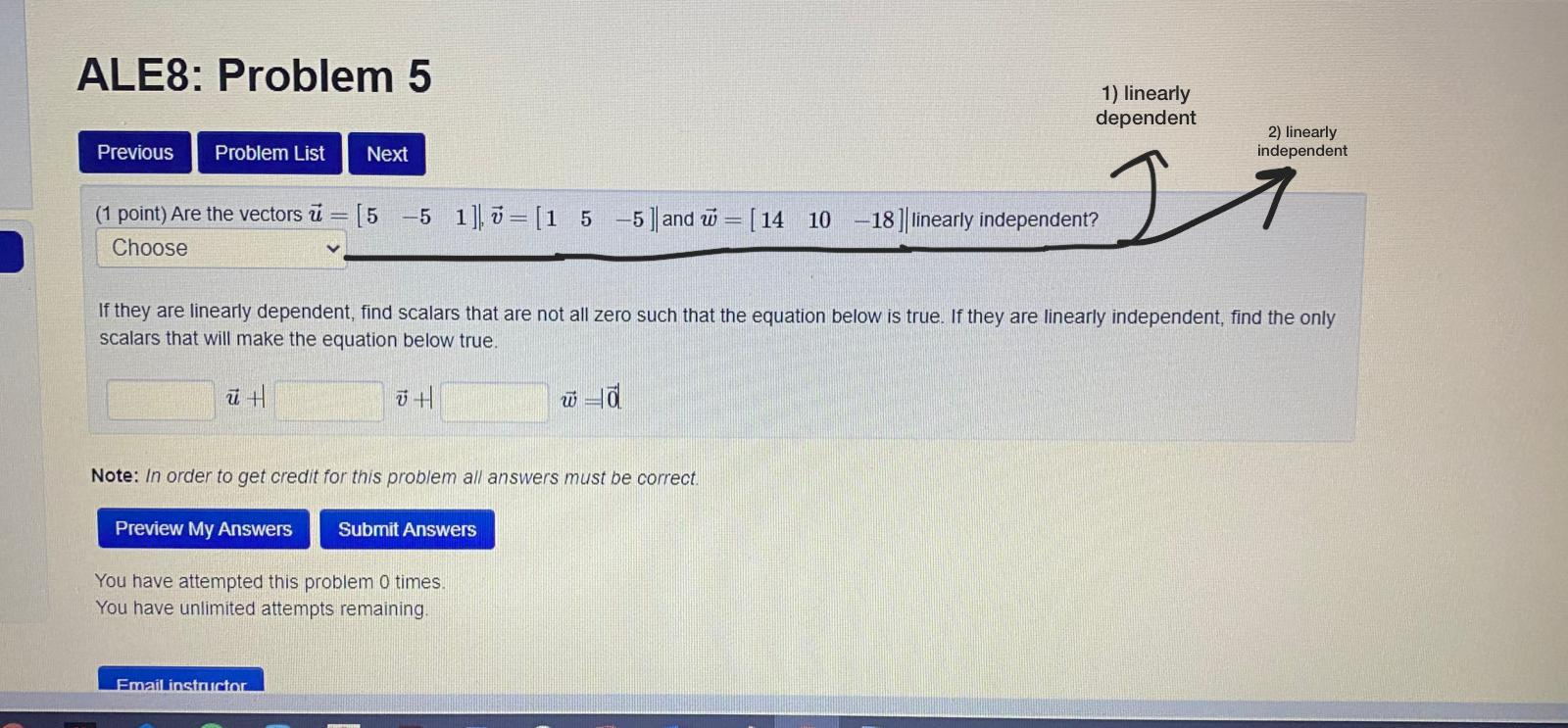### Create an Account

Already have account?

### Forgot Your Password ?

Home / Questions / ALES: Problem 5 1) linearly dependent 2) linearly independent Previous Problem List Next (...

# ALES: Problem 5 1) linearly dependent 2) linearly independent Previous Problem List Next (1 point) Are the vectors ū = [5 -5 1], v = (1 5 –5 ] and = [14 10 Choose I 18 ] linearly independent? If they

ALES: Problem 5 1) linearly dependent 2) linearly independent Previous Problem List Next (1 point) Are the vectors ū = [5 -5 1], v = (1 5 –5 ] and = [14 10 Choose I 18 ] linearly independent? If they are linearly dependent, find scalars that are not all zero such that the equation below is true. If they are linearly independent, find the only scalars that will make the equation below true. ūH ū+ ū la Note: In order to get credit for this problem all answers must be correct. Preview My Answers Submit Answers You have attempted this problem 0 times. You have unlimited attempts remaining, Email instructorApr 15 2021 View more View Less

#### Answer (Solved)Subscribe To Get Solution